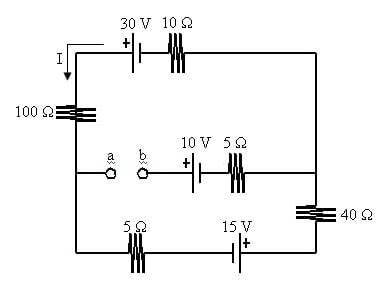# Potential Difference between two points of a circuit

## Homework Statement

Given the following circuit, what is Vab? Explain...## Homework Equations

Kirchoff's Law and Ohm's Law

## The Attempt at a Solution

Here is my solution... Is this describing it correctly?

Kirchoff’s Loop Law to find current:
-10 Ω (I) + 30 Ω V – 100 Ω (I) – 5 Ω (I) + 15 V – 40 Ω (I) = 0
45 V = 155 Ω (I)
I = 45 V / 155 Ω or .29 Amps

Potential Difference between points a and b:
1. At point a, we will assume there to be zero potential.
2. By going over the 5 Ω resistor, we lose 1.45 V (5 Ω * .29 A), thus leaving us at - 1.45 V.
3. The 15 V power supply adds 15 V, leaving us with 13.55 V.
4. The 40 Ω resistor takes away another 11.6 V (40 Ω * .29 A), leaving us with 1.95 V.
5. The 5 Ω resistor that we come in contact with next doesn’t take anything away as it doesn’t have current running through it, so we’re left with 1.95 V.
6. Now, we add another 10 V to the potential difference between the point a and point b due to the power supply.
7. We now have a combined Potential Difference between point a and point b to be 11.95 V.